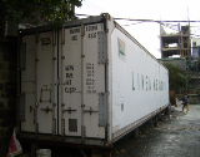How does our pricing work?Product Summary We charge \$2.30 every cubic foot, see details on how to determine cubic feet and special pricing.
Faq List

Pricing

Under 500 Cube: \$2.30

Over 500 Cube: \$1.75

Over 1000 Cube: \$1.50

To determine how many cubic feet you would like to send just multiply the length in inches x width in inches x height in inches then divide by 1728 to get cubic feet. If you are measuring in centimeters the formula is: multiply each centimeter by .39 to get inches. If you are measuring in cubic meters: divide each cubic meter by 35.5 to get cubic feet.Home | About Us | Services | Calendar | Project List | Employees | FAQs | Blog | Customer Feedback | Sitemap |